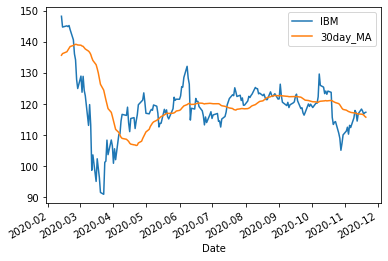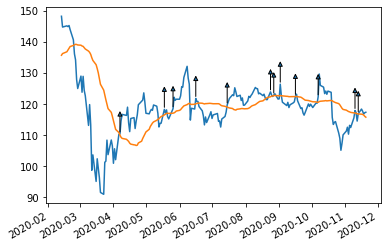# Price signals¶

Not a chartist by any stretch, but this application illustrates algorithmic approaches to trading by identifying moving average crossings as potential price signals. (A few more lines of codes suffice to identify crossings of different moving averages such as golden crosses.) One can connect those sorts of calculations with interactive broker's python API to complete the trading algorithm. There are oodles of libraries that do this, more, and do it better. The goal here is merely illustration.

In :
import pandas as pd # pandas is excellent for creating and manipulating dataframes, R-style
import numpy as np # great for simulations, we may not use for running regressions here
import matplotlib.pyplot as plt #graphing module with matlab-like properties
%matplotlib inline
import requests # to make requests to webpages (like mine)
import statsmodels.api as sm # module full of standard statistical and econometric models, including OLS and time-series stuff
from IPython.display import Latex # to be able to display latex in python command cells
import datetime


Let's get some daily closing price data for our good friend IBM. And then let's add a 30-day moving average. Let's also get rid of the 30-day burn-in data and plot the result.

In :
# get 10 years or so of adjusted close price data for IBM

end=datetime.datetime.today()
IBMdataF=IBMdata.to_frame() # converts the series format to a frame format which, for me, makes manipulations easier
IBMdataF['30day_MA'] = IBMdataF['IBM'].rolling(window=30).mean()
IBMdataF.dropna(subset=['30day_MA'], inplace=True)

IBMdataF.plot(y=['IBM','30day_MA'])

Out:
<matplotlib.axes._subplots.AxesSubplot at 0x21b37158af0>Now let's locate the signals and plot them on our chart. Again, if this was a proper buy signal we could feed it into an algorithmic trading routine using, say, Interactive Brokers' Python API.

In :
IBMdataF['Gap']=IBMdataF['IBM']-IBMdataF['30day_MA'] # positive if price is above 30 day MA
IBMdataF['GapLag']=IBMdataF.Gap.shift(1) # same previous trading day

IBMdataF['Signal']=np.where(IBMdataF.Gap < 0, False, (np.where(IBMdataF.GapLag < 0,True,False))) # a crossing happens where gap is negative yesterday but positive today

# let's mark buy signals with an arrow

fig= plt.figure()

ax.plot(IBMdataF.index,IBMdataF['IBM'])
ax.plot(IBMdataF.index,IBMdataF['30day_MA'])
fig.autofmt_xdate()

IBMdataBuy=IBMdataF[IBMdataF['Signal'] == True] # this just keeps the places where a buy signal is found

arrow_properties = dict(
facecolor="blue", width=0.1,

arrow_x = date
# print(arrow_x,arrow_y)

ax.annotate(
'', xy=(arrow_x, arrow_y),
xytext=(0, 25), textcoords='offset points',
arrowprops=dict(arrowstyle="<|-"))In :
IBMdataBuy

Out:
IBM 30day_MA Gap GapLag Signal
Date
2020-04-07 110.352898 109.967258 0.385639 -0.586294 True
2020-05-18 118.273903 116.477695 1.796208 -2.120724 True
2020-05-26 118.468498 117.031326 1.437172 -1.769947 True
2020-06-16 121.766853 120.057604 1.709249 -1.531374 True
2020-07-15 119.674973 119.000709 0.674264 -1.758154 True
2020-08-24 123.883621 122.307284 1.576337 -0.644110 True
2020-08-27 122.868347 122.655602 0.212745 -0.186710 True
2020-09-02 126.347885 122.665207 3.682678 -0.990453 True
2020-09-16 122.444496 122.350476 0.094020 -1.660332 True
2020-10-07 122.296638 120.523350 1.773289 -0.315424 True
2020-11-10 117.910004 117.103440 0.806564 -1.616821 True
2020-11-13 116.849998 116.783879 0.066120 -2.350434 True

Now in practice we would also want to back-test this "strategy." We would for instance expect that the mean return on the day following a buy signal is significantly higher than on other days. So we are asking: assume I buy at the close of crossing days and sell at the close of the next day, do I generate an average return that is significantly different from the return I average by buying on other days?

Proper financial tests take a number of other factors into account but, once again for illustration, we will perform a simple mean test. For that, we begin by downloading longer data and then proceed as before. Not surprisingly given the naivete of our approach, we don't get much of a t-stat and and we get high p-value. We can't reject the hypothesis that returns are the same on average on buy days than on other days with much confidence. Worse, we get a negative return on average. So this notebook is not going to make us rich.

In :
from scipy.stats import ttest_ind

end=datetime.datetime.today()
IBMdataF=IBMdata.to_frame() # converts the series format to a frame format which, for me, makes manipulations easier
IBMdataF['30day_MA'] = IBMdataF['IBM'].rolling(window=30).mean()
IBMdataF.dropna(subset=['30day_MA'], inplace=True)

IBMdataF['Gap']=IBMdataF['IBM']-IBMdataF['30day_MA'] # positive if price is above 30 day MA
IBMdataF['GapLag']=IBMdataF.Gap.shift(1) # same previous trading day

IBMdataF['Signal']=np.where(IBMdataF.Gap < 0, False, (np.where(IBMdataF.GapLag < 0,True,False))) # a crossing happens where gap is negative yesterday but positive today

IBMdataF['rIBM']=-np.log(IBMdataF['IBM'])+np.log(IBMdataF.IBM.shift(-1)) #computes the one day-ahead return based on adjusted series for IBM

IBMdataF.dropna(subset=['rIBM'], inplace=True)


Ttest_indResult(statistic=-0.8251997487849901, pvalue=0.40933082734760695)